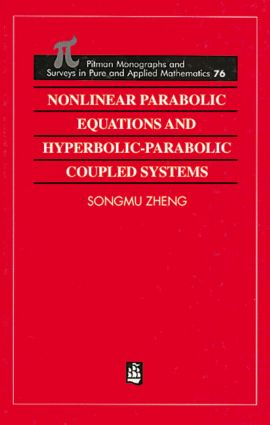Nonlinear Parabolic Equations and Hyperbolic-Parabolic Coupled Systems

1st Edition

Chapman and Hall/CRC

272 pages

Purchasing Options:\$ = USD
Hardback: 9780582244887
pub: 1995-08-08
SAVE ~\$48.00
\$240.00
\$192.00
x

FREE Standard Shipping!

Description

This monograph is devoted to the global existence, uniqueness and asymptotic behaviour of smooth solutions to both initial value problems and initial boundary value problems for nonlinear parabolic equations and hyperbolic parabolic coupled systems. Most of the material is based on recent research carried out by the author and his collaborators.

The book can be divided into two parts. In the first part, the results on decay of solutions to nonlinear parabolic equations and hyperbolic parabolic coupled systems are obtained, and a chapter is devoted to the global existence of small smooth solutions to fully nonlinear parabolic equations and quasilinear hyperbolic parabolic coupled systems. Applications of the results to nonlinear thermoelasticity and fluid dynamics are also shown.

Some nonlinear parabolic equations and coupled systems arising from the study of phase transitions are investigated in the second part of the book. The global existence, uniqueness and asymptotic behaviour of smooth solutions with arbitrary initial data are obtained. The final chapter is further devoted to related topics: multiplicity of equilibria and the existence of a global attractor, inertial manifold and inertial set.

A knowledge of partial differential equations and Sobolev spaces is assumed. As an aid to the reader, the related concepts and results are collected and the relevant references given in the first

chapter. The work will be of interest to researchers and graduate students in pure and applied mathematics, mathematical physics and applied sciences.

Preface

Preliminaries

Decay of solutions to linear evolution equations

Global existence for small initial data

Global existence for large initial data

Stationary problems and asymptotic behaviour

Bibliography

Index

Zheng\, Songmu